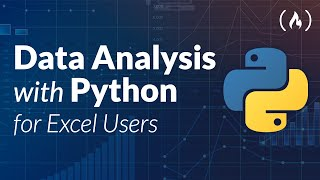# Data Analysis with Python for Excel Users - Full Course

Learn how to use Python and Pandas for data analysis. This course will be especially helpful if you have experience with Excel, but that is not required. Learn how to create pivot tables, work with data, and make visualizations using Python, Pandas, and Jupyter Notebook.

💻 Source Code & Data...
Learn how to use Python and Pandas for data analysis. This course will be especially helpful if you have experience with Excel, but that is not required. Learn how to create pivot tables, work with data, and make visualizations using Python, Pandas, and Jupyter Notebook.

💻 Source Code & Datasets: https://github.com/ifrankandrade/python-course-for-excel-users

✏️ Course developed by Frank Andrade.
🔗 PDF Python Cheat Sheet for this Course: https://frankandrade.ck.page/d3b1761715
🔗 My Complete Python Courses for Data Analysis & Data Science: https://www.udemy.com/user/frank-andrade-13/

⭐️ Course Contents ⭐️
⌨️ (0:00:00) Intro
⌨️ (0:01:48) Install Python and Jupyter Notebook with Anaconda
⌨️ (0:03:53) Jupyter Notebook Interface
⌨️ (0:13:56) Cell Types and Cell Mode
⌨️ (0:21:34) Jupyter Notebook Shortcuts
⌨️ (0:26:39) Module 1 - Hello World
⌨️ (0:30:30) Data Type
⌨️ (0:39:10) Variables
⌨️ (0:46:53) Lists
⌨️ (1:11:14) Dictionaries
⌨️ (1:21:50) IF Statement
⌨️ (1:28:04) FOR Loop
⌨️ (1:33:49) Functions
⌨️ (1:40:59) Modules
⌨️ (1:44:41) Module 2 -Introduction to Pandas
⌨️ (1:51:08) How to create a dataframe
⌨️ (2:07:34) How to show a dataframe
⌨️ (2:14:24) Basic Attributes, Functions and Methods
⌨️ (2:26:17) Selecting One Column from a Dataframe
⌨️ (2:32:13) Selecting Two or More Columns from a Dataframe
⌨️ (2:37:50) Add New Column to a Dataframe (Simple Assignment)
⌨️ (2:47:51) Operations in dataframes
⌨️ (2:56:04) The value_counts() method
⌨️ (3:00:16) Sort a DataFrame with the sort_values() method
⌨️ (3:09:56) Module 3: Introduction to Pivot Tables in Pandas
⌨️ (3:14:42) The pivot() method
⌨️ (3:20:49) The pivot_table() method
⌨️ (3:29:00) Data Visualization with Pandas (New Dataset + Pivot Table)
⌨️ (3:38:38) Lineplot
⌨️ (3:43:05) Barplot
⌨️ (3:50:52) Piechart
⌨️ (3:54:36) Save Plot and Export Pivot Table

🎉 Thanks to our Champion and Sponsor supporters:
👾 Raymond Odero
👾 Agustín Kussrow
👾 aldo ferretti
👾 Otis Morgan
👾 DeezMaster

--

Learn to code for free and get a developer job: https://www.freecodecamp.org

Read hundreds of articles on programming: https://freecodecamp.org/news